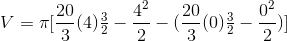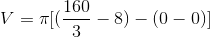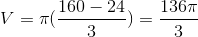# AP Calculus AB : How to find volume of a region

## Example Questions

### Example Question #1 : How To Find Volume Of A Region

Consider the region bounded by the-axis, the line, and the function, where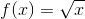.  Find the volume of the solid generated by rotating the region described about the line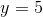.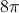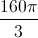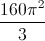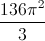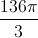Explanation:

To find the volume, one would use the washer method: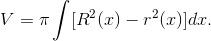For this problem: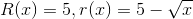.  So, the following integral would give the volume: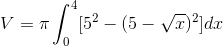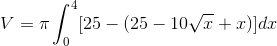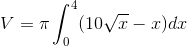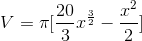, evaluated fromto.